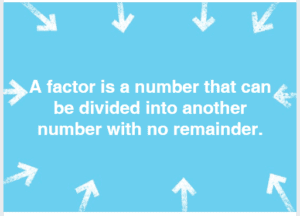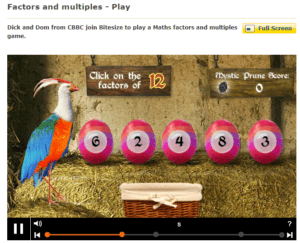Hide

Grammar School Bucks Entrance Exams, Maths and Verbal Reasoning - Week 5

Welcome to week 5 of my interactive 11+ blog for Bucks children.

This week I am looking at multiples, factors, another Verbal Reasoning technique and some essential vocabulary to add to your growing knowledge. It includes exclusive example materials, based on real 11+ style questions.A multiple is the answer you get when two numbers are multiplied.

Lowest common multiple (LCM): and what is it for?

Eg LCM of 10 and 25

The multiples of 10 are: 10, 20, 30, 40, 50, 60, 70, 80, 90, 100. The multiples of 25 are: 25, 50, 75, 100

Multiples they have 'in common' are 50 and 100 50 100 So the LCM is 50

Here's a clip.

This is useful for finding the lowest common denominator of two fractions. (more of this in later weeks.)

1. Find the LCM of 8 and 7.
List the multiples of 8. __________________ List the multiples of 7. __________________
List the common multiples. __________________ What is the LCM? __________________

2. Find the LCM of 15 and 20.
List the multiples of 15. __________________ List the multiples of 20. __________________
List the common multiples. __________________ What is the LCM? ___________________

3. Find the LCM of 21 and 35.
List the multiples of 21. __________________ List the multiples of 35. __________________
List the common multiples. __________________ What is the LCM? ___________________

4. Find the LCM of 6 and 18.
List the multiples of 6. __________________ List the multiples of 8. __________________
List the common multiples. __________________ What is the LCM? ___________________

5. Find the LCM of 10 and 11.
List the multiples of 10. __________________ List the multiples of 11. __________________
List the common multiples. __________________ What is the LCM? __________________

6. Find the LCM of 2 and 12.
List the multiples of 2. __________________ List the multiples of 12. __________________
List the common multiples. __________________ What is the LCM? ___________________

7. Find the LCM of 3 and 9.
List the multiples of 3. __________________ List the multiples of 9. __________________
List the common multiples. __________________ What is the LCM? ___________________

8. Find the LCM of 7 and 5.
List the multiples of 7. __________________ List the multiples of 5. __________________
List the common multiples. __________________ What is the LCM? ___________________A factor is a number that divides into a number.

It is useful to be able to quickly work out the highest common factor of numbers when we are simplifying.

For Greatest Common Factor, (GCF)

Find the GCF of 12 and 20

List the factors of 12: 12, 1, 6, 2, 3, 4________ List the factors of 20: 10, 2, 5, 4.

Find the common factors 2, 4 GCF is 4

1. Find the GCF of 8 and 12.
List the factors of 8. __________________ List the factors of 12. __________________
List the common factors. __________________ What is the GCF? __________________
2. Find the GCF of 15 and 20.
List the factors of 15. __________________ List the factors of 20. __________________
List the common factors. __________________ What is the GCF? ___________________
3. Find the GCF of 21 and 35.
List the factors of 21. __________________ List the factors of 35. __________________
List the common factors. __________________ What is the GCF? ___________________
4. Find the GCF of 6 and 18.
List the factors of 6. __________________ List the factors of 18. __________________
List the common factors. __________________ What is the GCF? ___________________

5 Find the GCF of 6 and 3.
List the factors of 6. __________________ List the factors of 3. __________________
List the common factors. __________________ What is the GCF? __________________
6. Find the GCF of 14 and 21.
List the factors of 14. __________________ List the factors of 21. __________________
List the common factors. __________________ What is the GCF? ___________________
7. Find the GCF of 64 and 18.
List the factors of 64. __________________ List the factors of 18. __________________
List the common factors. __________________ What is the GCF? ___________________
8. Find the GCF of 9 and 45.
List the factors of 9. __________________ List the factors of 45. __________________
List the common factors. __________________ What is the GCF? ___________________http://www.bbc.co.uk/bitesize/ks2/maths/number/factors_multiples/play/

Primes are numbers that don't appear in the times tables, except the one times tables. It is useful to know your primes because you can't cancel down or simplify prime numbers: when you reach a prime, you know it is time to stop. Here's a reminder of your primes. You need to learn these.The link below covers Primes, LCM, HCF. Do all pages, and the test section.If you still don't get it, look at the links below.

https://www.mathsisfun.com/greatest-common-factor.html

https://www.mathsisfun.com/least-common-multiple.html

Verbal Reasoning

Find the missing word.

Example: The LIEST I can be there is noon becomes the EARliest I can be there is noon.

The three letter word was EAR.

Here's another example:

Do your work CFULLY. What word 'fits' that sentence? It has to be three letters long and go somewhere in the word CFULLY.

The word ends in ly so is probably an adverb, a word that describes a verb.

C---FULLY

I'll give you a clue, it goes in the gap there. So you know the three-letter-word does not always go right at the beginning of the word in capitals.

CAREFULLY

The word is ARE. (It doesn't have to have the same pronunciation in the long word and in the short word.)

Here's some for practice:

1) Her HING is getting worse as she gets older.

2) She said her FAVRITE food was cheese.

3) The PERMER loved applause.

4) My HBRUSH is broken..

5) My shoes are KING me.

6) WHATR goes around, comes around.

7) I HD that you were doing well.

8) What OCATION would you like when you grow up?

9) The girl WPERED a wish.

10) The puppy WPERED for its mum.

11) That is an IMPROVET on your first effort.

This weeks 10(ish) words:

amble, stroll, saunter, mosey, ramble, meander, dawdle, wander, (all mean to walk at a slow pace)

march, trek, hike, stride, (all mean to walk a medium pace)

trudge, plod, slog, (all mean to walk with difficulty)

run, jog, race, sprint, dart, dash, hurry, canter, gallop, (to move quickly)

NEXT WEEK -
Verbal Reasoning: Find the Hidden Word
Maths: Square Numbers, Triangular Numbers, and Fractions.